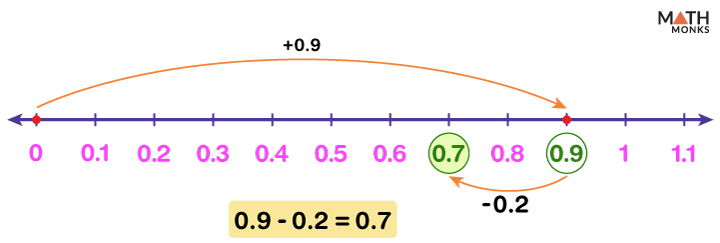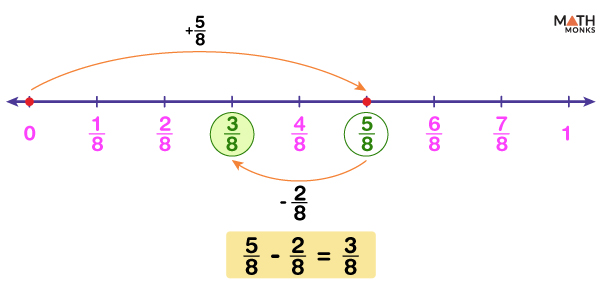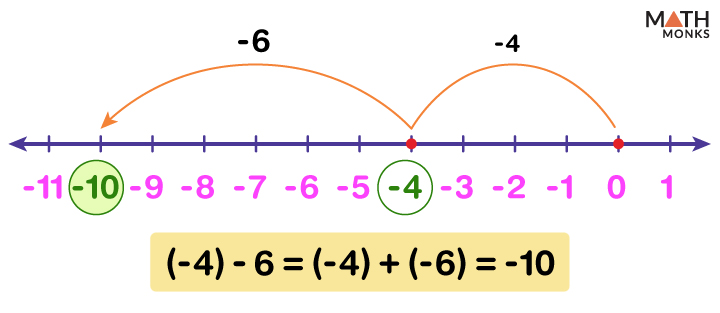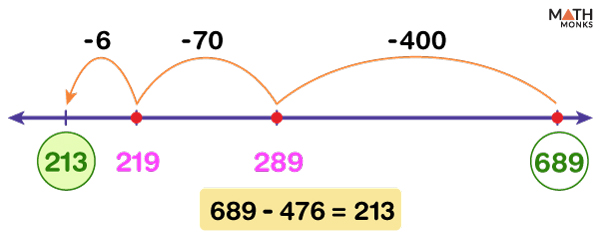# Subtraction on a Number Line

Subtraction on a number line helps us to find the difference between two numbers easily, given the numbers are small. These include integers, fractions, and decimal numbers. It also helps us to locate the result of a subtraction problem.

The three main parts of a subtraction problem are:

1. Minuend: The number from which we subtract
2. Subtrahend: The number that we subtract from the minuend
3. Difference: The result of subtraction

For example, 10 – 3 gives 7

To perform subtraction, we first locate the minuend on the number line. Then we move back the number of steps based on the value of the subtrahend.

There can be three such cases:

## Positive Number from a Positive Number

Subtracting a positive number from a positive number is just a normal subtraction. In the above example, 10 – 3 gives 7. Here, 10 is the minuend, 3 is the subtrahend, while 7 is the difference or the result of subtraction.

As the minuend 10 is a positive number, we move 10 steps to the right of 0.

Similarly, the subtrahend 3 is also positive; thus, we move 3 steps to the left of 10.

Find the value of 15 – 6 using a number line.

Solution:Here, minuend = 15 and subtrahend = 6
We first locate 15 on the number line and then move 6 steps to the left of 15.
Thus the answer is 15 – 6 = 9
Like integers, we can also subtract decimals and fractions on a number line.

### Subtracting Decimals

Subtract 0.2 from 0.9 using a number line.

Solution:Here, minuend = 0.9 and subtrahend = 0.2
First, we divided the range 0 and 1 into 10 equal parts on a number line.
Then we located 0.9 on the number line and then moved 2 steps to the left of 0.9
Thus, we get the answer 0.9 – 0.2 = 0.7

### Subtracting Fractions

Subtract ${\dfrac{2}{8}}$ from ${\dfrac{5}{8}}$ using a number line.

Solution:Here, minuend = ${\dfrac{5}{8}}$ and subtrahend = ${\dfrac{2}{8}}$
We first divided the region between 0 and 1 into eight equal parts on a number line.
Then we located ${\dfrac{5}{8}}$ on the number line and then moved 2 steps to the left of${\dfrac{5}{8}}$
Thus the answer is ${\dfrac{5}{8}}$ –${\dfrac{2}{8}}$ = ${\dfrac{3}{8}}$

## Positive Number from a Negative Number

While subtracting a positive number from a negative number, the operation turns into the addition between those two numbers, and the sign becomes negative. While subtracting two numbers, we can change the sign of the subtrahend and follow the addition rules.

For example, (-12) – 2 = (-14). Here, (-12) is the minuend, 2 is the subtrahend, while (-14) is the difference or the result.

Now (-12) – 2 is written as (-12) + (- 2)

(-12) being a negative number, we move 12 steps to the left of 0.

Finally, (- 2) being negative, we move 2 more steps to the left side of (-12) to get the result.

Subtract 5 from -13 using a number line.

Solution:Here, minuend = (-4) and subtrahend = 6, (-4) – 6 = (-4) + (-6)
We first located (-4) on the number line and then moved 6 steps to the left of (-4) to get the result.
Thus, we get the answer (-4) – 6 = (-10)

## Negative Number from a Negative Number

Subtraction of a negative number from another negative number turns into addition, an addition operation. Thus subtracting a negative number is the same as adding the absolute value of that number. For example,

(-9) – (-4) = (-5). Here, (-9) is the minuend, (-4) is the subtrahend, while (-5) is the difference or the result.

Now (-9) – (-4) is written as (-9) + 4

As (-9) is a negative number, we move 9 steps to the left of 0.

Next, 4 being a positive number, we move 4 steps to the right side from (-9) to get the answer.

Thus, we get the answer (-5)

Subtract (-3) from (-7) using a number line.

Solution:Here, minuend = (-7) and subtrahend = (-3)
As we know, subtracting a negative number is the same as adding the absolute value of that number.
(-7) – (-3) = (-7) + 3
Now we followed the addition rule.
We first located (-7) on the number line and then moved 3 steps to the right of (-7).
Thus the answer is (-7) – (-3) = (-7) + 3 = (-4).

## Subtraction on an Open Number Line

For 2-digit and 3-digit subtraction, we use an open number line with no numbers marked on it.

### 2-Digit subtraction

For example, 55 – 34 gives 21. Here, 55 is the minuend, 34 is the subtrahend, while 21 is the difference or the result.

As minuend 55 is a positive number, we mark it on an open number line to the right side of 0.

Next, we count the number of tens and ones in the subtrahend, i.e., 34.

34 = (3 × 10) + (4 × 1)

Finally, we skip count back 3 times by 10s and then 4 times by 1s and mark the numbers (45, 35, 25,…., 21) simultaneously on the number line after every jump.

Thus the result is 55 – 34 = 21.

Calculate 84 – 56 using a number line.

Solution:Here, minuend = 64 and subtrahend = 36.
First, we mark the minuend 64 on an open number line.
Subtrahend 36 = (3 ×10) + (6 × 1),
So we took 3 longer jumps to count back by 10s and 6 shorter jumps to count back by 1s and mark the numbers (54, 44, 34,…., 28) simultaneously on the number line after every jump.
Thus we get the result 64 – 36 = 28.

### 3 -Digit Subtraction

Like 2-digit subtraction, we can also perform 3-digit subtractions using an open number line.

For example, 390 – 259 gives 231. Here, 390 is the minuend, 259 is the subtrahend, and 131 is the difference or the result.

As minuend 390 is a positive number, we mark it on an open number line to the right side of 0.

Next, we count the number of hundreds, tens, and ones in the subtrahend, i.e., 259.

259 = (2 × 100) + (5 × 10) + (9 × 1)

Now we take 2 longer jumps to count back 100s, 5 moderate jumps to count back 10s, and 9 shorter jumps to count back 1s. We can also skip count to show all the jumps and mark the numbers (190, 140, 131) simultaneously on the number line after every jump.

Thus we get the result 390 – 259 = 131

Find out the value of 689 – 476 using a number line.

Solution:Here, minuend = 689 and subtrahend = 476.
First, we mark the minuend 689 on an open number line.
As subtrahend 476 = (4 × 100) + (7 × 10) + (6 × 1), we take 4 longer jumps to count back 100s, 7 moderate jumps to count back 10s, and 6 shorter jumps to count back 1s. Each number (289, 219, 213) is marked simultaneously after every jump on the number line.
Thus we get the result 689 – 476 = 213

## Rules for Subtracting Two Numbers

Let us summarize the rules for subtracting two numbers below. We denote minuend by M and subtrahend by S.